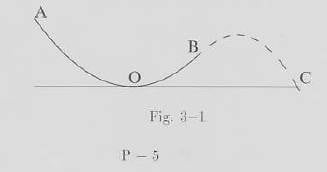# Parabolic motion along a frictionless path

## Homework Statement

A sufficiently small steel smooth spherical object is initially at rest at point A on the smooth surface of a finite parabolic curve AOB in a vertical plane as shown in Fig.3-1, where the point O stands for the bottom point, the tangential line at O being horizontal, the vertical height at point A (relative to O) being 2H, the vertical height at point B (relative to O) being H, the horizontal distance between B and O being 2H. At time t=0, the object is released slowly to move down frictionlessly along the curve toward point B, where it departs into the air. The effects of the rotation of the object around its center is assumed to be negligible.(1) The slope angle of the object orbit measured from the horizontal level just after release in the air at B is

(2)Finally the object will reach a point C with the same horizontal level as that at O. The necessary time to travel to C from B is

## The Attempt at a Solution

For the question1, I got the speed at point B = square root of 2gH. Then I named the angle which question asked, θ, so I got speed in y-axis and x-axis, (square root of 2gH)sinθ and (square root of 2gH)cosθ ,respectively. And I tried to find the θ by combined the 2 speed into triangle picture but it was not a correct way to find the answer.
(I only know that the correct answer is 45°)

For the question2, I used a projectile range's formula R=v^2sin2θ/g and I got R=2H. From S=vt (x-axis), 2H= (square root of 2gH)cos45°t, then I got time = (square root of H/g)*2 but it was wrong answer because the correct answer is (1+square root of 3)*square root of H/g.

#### Attachments

Last edited by a moderator:

PeterO
Homework Helper

## Homework Statement

A sufficiently small steel smooth spherical object is initially at rest at point A on the smooth surface of a finite parabolic curve AOB in a vertical plane as shown in Fig.3-1, where the point O stands for the bottom point, the tangential line at O being horizontal, the vertical height at point A (relative to O) being 2H, the vertical height at point B (relative to O) being H, the horizontal distance between B and O being 2H. At time t=0, the object is released slowly to move down frictionlessly along the curve toward point B, where it departs into the air. The effects of the rotation of the object around its center is assumed to be negligible.
View attachment 225802

(1) The slope angle of the object orbit measured from the horizontal level just after release in the air at B is

(2)Finally the object will reach a point C with the same horizontal level as that at O. The necessary time to travel to C from B is

## The Attempt at a Solution

For the question1, I got the speed at point B = square root of 2gH. Then I named the angle which question asked, θ, so I got speed in y-axis and x-axis, (square root of 2gH)sinθ and (square root of 2gH)cosθ ,respectively. And I tried to find the θ by combined the 2 speed into triangle picture but it was not a correct way to find the answer.
(I only know that the correct answer is 45°)

For the question2, I used a projectile range's formula R=v^2sin2θ/g and I got R=2H. From S=vt (x-axis), 2H= (square root of 2gH)cos45°t, then I got time = (square root of H/g)*2 but it was wrong answer because the correct answer is (1+square root of 3)*square root of H/g.
For part (1), I would be making use of the fact the AOB path is part of a parabola.
Comparing it to the y = ax^2 parabola, we can use the horizontal distance from 0 to B, and the vertical distance from O to B to find out the value of a, and then calculus to find the track angle.

rude man
Homework Helper
Gold Member
Question 1: from vB2 = vB2cos2θ + vB2sin2θ just gives an identity without being able to solve for θ.
But you can use vx^2 + vy^2 = vB2 by combining with the slope of the parabola at point B.

Question 2 is elementary since the sphere is no longer constrained by the parabolic track.

Question 1: from vB2 = vB2cos2θ + vB2sin2θ just gives an identity without being able to solve for θ.
But you can use vx^2 + vy^2 = vB2 by combining with the slope of the parabola at point B.

Question 2 is elementary since the sphere is no longer constrained by the parabolic track.

I don’t understand question2, could you explain more?

rude man
Homework Helper
Gold Member
I don’t understand question2, could you explain more?
You know the initial height "above ground" and initial x and y velocities of a launched mass, and you're asked for the time to "hit the ground"; this is a very elementary problem you should be able to handle even with high school physics.

(A MUCH more difficult problem would have been to compute the time from A to O or O to C since for that portion of the trajectory there are more forces than just gravity acting on the sphere.)

You know the initial height "above ground" and initial x and y velocities of a launched mass, and you're asked for the time to "hit the ground"; this is a very elementary problem you should be able to handle even with high school physics.

I got the answer now by calculating y-axis with S=ut+1/2at^2. But I don’t know why my first method was wrong. I hope you could explain me.
Thanks

haruspex
•